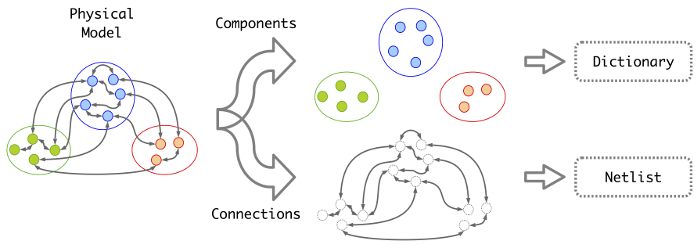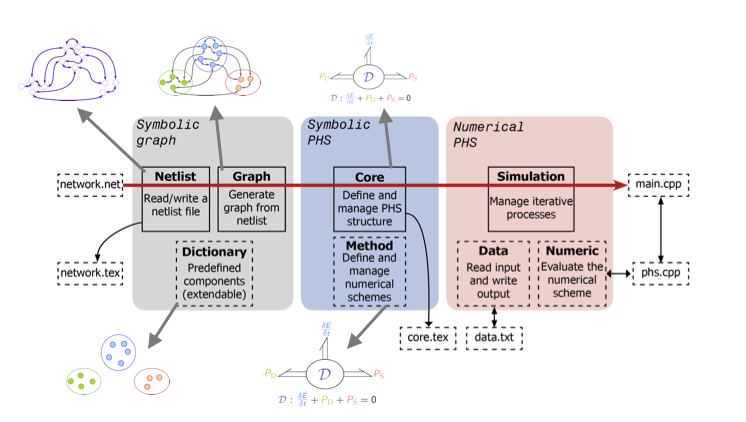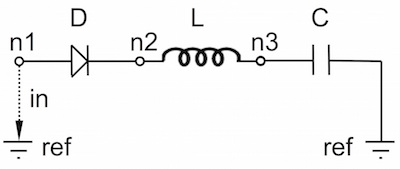# PyPHS

A Python software (Py) dedicated to the simulation of multi-physical Port-Hamiltonian Systems (PHS) described by graph structures.

The PHS formalism decomposes network systems into conservative parts, dissipative parts and source parts, which are combined according to an energy conserving interconnection. This approach permits to formulate the dynamics of multi-physical systems as a set of differential-algebraic equations structured according to energy flows. This structure proves the passivity of the system, including the nonlinear cases. Moreover, it guarantees the stability of the numerical simulations for an adapted structure preserving numerical method.The main objects of the library are introduced in this presentation. The standard workflow is as follows.

1. Inputs are netlist descriptions of network systems (very similar to SPICE netlists).
2. The associated graphs are analyzed to produce the core system's dynamics equations in the PHS formalism.
3. Simulations (i.e. numerical solving of DAE equations) are performed based on a variety of numerical methods (can be extended with new ones).
4. The corresponding C++ simulation code is automatically generated and called from python (can also be used in bigger applications).
5. LaTeX description files can be generated (for documentation, publication, etc.).• The Python class Core defines symbolically a continuous-time Port-Hamiltonian structure.
• The Python class Method defines symbolically a discrete-time port-Hamiltonian structure derived from a given Core object and for several numerical schemes. It includes a structure preserving numerical method (see [NumericalMethod2015]).
• The Python class Netlist reads and writes the descriptions of network systems.
• The Python class Graph defines a network structure for the automated generation of Core from Netlist, based on (i) the implementation of a specially designed graph analysis (see [GraphAnalysis2016]), and (ii) a set of elementary components compiled in the Dictionary.
• The Python class Simulation evaluates iteratively a given Method object to produce the data result in text files. The evaluation can run in pure Python code with the Numerical object, or can run in C++ through the generated C++ files.

## Status

This package is in development status Beta. The continuous integration is checked with Travis for Unix systems and AppVeyor for Windows systems (see build status below).

## Licence

PyPHS is distributed under the french CeCILL-B licence.

## Installation

It is possible to install PyPHS from package (if you just want to use it) or source (if you plan to use it for development). Whichever method you choose, make sure that all prerequisites are installed.

### Python prerequisites

The PyPHS package run on Python 2.7 and Python 3.5 or newer (3.4 is no longer tested), with the following packages installed:

Please refer to the requirements.txt file for the required versions and make sure that these modules are up to date.

Additionally, theano is used if it can be found on the system, for faster numerical evaluation of symbolic expressions.

### C++ prerequisites

The generated C++ sources build with CMake >= 3.1 (see Configuration below). The code relies on the Eigen library (not needed for pure Python usage).

### Install from package

The easiest way to install the package is via pip from the PyPI (Python Package Index):

pip install pyphs


This includes the latest code and should install all dependencies automatically. If it complains about some missing dependencies, install them the same way with pip beforehand.

You might need higher privileges (use su or sudo) to install the package globally. Alternatively you can install the package locally (i.e. only for you) by adding the --user argument:

pip install --user pyphs


### Install from source

If you plan to use the package as a developer, clone the Git repository:

git clone --recursive https://github.com/pyphs/pyphs.git


Then you can simply install the package in development mode:

python setup.py develop --user


To run the included tests:

python setup.py test


### Configuration

After installation, it is recommended to configure the config.py to your needs. Particularly, this is where the local path to the CMake binary and Eigen library is specified.

Your local config.py file is located at the root of the PyPHS package, which can be recovered in a Python interpreter with

from pyphs import path_to_configuration_file
print(path_to_configuration_file)


### Upgrade of existing installations

To upgrade the package, please use the same mechanism (pip vs. source) as you did for installation.

#### Upgrade a package

First, manually uninstall the package:

pip uninstall pyphs


and reinstall as explained above.

#### Upgrade from source

Pull the latest sources:

git pull


## Package structure

The package is divided into the following folders:

/pyphs/tutorials
Tutorials for the main PyPHS classes (executable programs).
/pyphs/examples
Various real-life applications (executable programs).
/pyphs/core
Core class :
This is the central object of the PyPHS package. It implements the core PHS structure and provides several methods for its manipulation (reorganization, connection, simplification, etc.).
/pyphs/graphs
Netlist class :
Management of netlist description files.
Graph class :
1. Construction and manipulation of network systems,
2. Analysis of network realizability,
3. Generation of PHS equations (Core).
/pyphs/dictionary
• Components are Graph objects.
• The dictionary is organized in thematic sub-packages (electronics, thermics, fractional calculus, etc.).
• Each theme is organized in component sub-packages (electronics.resistor, thermics.transfer, fraccalc.fracderec, etc.).
/pyphs/numerics
Evaluation class :
Numerical evaluation of a given Core.
Method object :
Construction of the symbolic expressions associated with several numerical methods (theta-schemes, trapezoidal rule, discret gradient, etc.).
Simulation object :
Manage the iterative evaluation and associated results data for a given Method.
Numeric object :
Python evaluation of a given Method.
Data object :
Methods for writing, reading and rendering Simulation file results.
/pyphs/tests
Test programs executed by nose (see above).
/pyphs/misc
Miscellaneous tools (plots, LaTeX code generation, signal processing, files I/O).

## Documentation

Most of the documentation can be found in the website. In particular, you can see the two following resources:

• The slides from a talk given at IRCAM that introduces most the scientific background.
• The tutorial that shows practical usage of most PyPHS objects (3Mb).

### Theoretical overview

The development of PyPHS started as an implementation of the methods proposed in the reference [GraphAnalysis2016], in which the port-Hamiltonian formalism, the graph analysis and the structure preserving numerical method are exposed. This is worth to read before using the package.

### Q&A Mailing list

The package mailing list is at https://groups.google.com/forum/#!forum/pyphs.

### Tutorials and examples

The package comes with a set of tutorials for the use of the main functionalities (definition, evaluation, and simulation of a core PHS structure). More tutorials are to come. Additionally, you can see the examples scripts. Both the tutorials and the examples folders are located at your package root, which can be recovered in Python interpreter with

from pyphs import path_to_examples, path_to_tutorials
print(path_to_examples)
print(path_to_tutorials)


## Typical use

Consider the following serial diode-inductor-capacitor (DLC) electronic circuit:with the following physical parameters:

 Parameter Description (SI unit) Typical value Is Diode saturation current (A) 2e-9 v0 Diode thermal voltage (V) 26e-3 mu Diode ideality factor (dimensionless) 1.7 R Diode connectors resistance (Ohms) 0.5 L Inductance value (H) 0.05 C Capacitance value (F) 2e-06

### 1. Define the Netlist

Put the following content in a text file with .net extension, (here dlc.net):

electronics.source in ('#', 'n1'): type='voltage';
electronics.diode D ('n1', 'n2'): Is=('Is', 2e-9); v0=('v0', 26e-3); mu=('mu', 1.7); R=('Rd', 0.5);
electronics.inductor L ('n2', 'n3'): L=('L', 0.05);
electronics.capacitor C ('n3', '#'): C=('C', 2e-06);

### 2. Perform graph analysis

Run the following in a Python interpreter in the netlist file directory:

import pyphs as phs

# Read the 'dlc_netlist.net'
netlist = phs.Netlist('dlc.net')

# Construct the graph associated with 'netlist'
graph = netlist.to_graph()

# Construct the core Port-Hamiltonian System from 'graph'
core = graph.to_core()


### 3. Export LaTeX

# Add netlist to LaTeX content
content = phs.netlist2tex(netlist)

# Add PHS core to LaTeX content
content += phs.core2tex(core)

# Write ready-to-use .tex document
phs.texdocument(content,
title='DLC',
path='dlc.tex')


This yields the following tex file:

which is compiled to produce the following pdf file:

### 4. Export C++ code

# Numerical method for time discretization of 'core'
# with default configuration
method = core.to_method()

# Export the set of C++ file for simulation
method.to_cpp()


This yields the following cpp files:

The core.h defines a class of DLC systems with an update method to be called at each iteration for the simulations.

## Authors and Affiliations

PyPHS is mainly developed by Antoine Falaize and Thomas Hélie, respectively in

See the AUTHORS file for the complete list of authors.

## Short History

PyPHS was initially developed between 2012 and 2016 as a part of the PhD thesis of Antoine Falaize under the direction of Thomas Hélie, through a funding from the French doctoral school EDITE (UPMC ED-130) and in connection with the French National Research Agency project HaMecMoPSys.